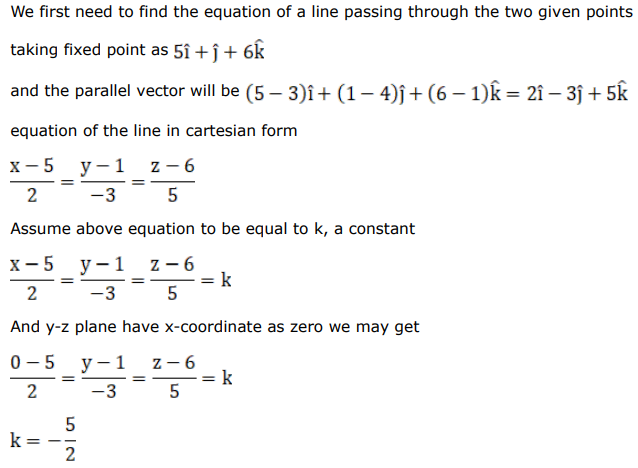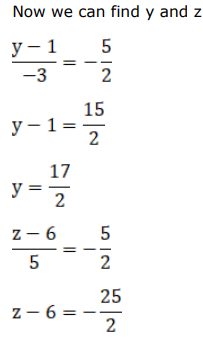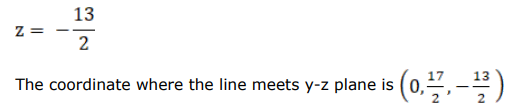# The coordinates of the point where the line through the pointsQuestion:

The coordinates of the point where the line through the points $A(5,1,6)$ and $B(3,4,1)$ crosses the yz-plane is

A. $(0,17,-13)$

B. $\left(0, \frac{-17}{2}, \frac{13}{2}\right)$

C. $\left(0, \frac{17}{2}, \frac{-13}{2}\right)$

D. none of these

Solution: